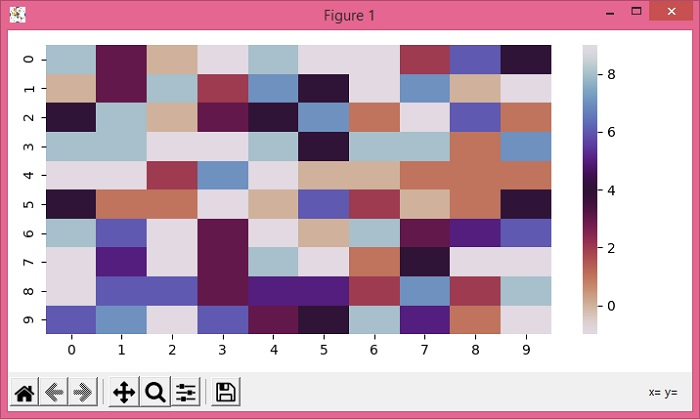# How to make a heatmap square in Seaborn FacetGrid using Matplotlib?

To make a heatmap square in Seaborn facetgrid, we cn use heatmap() method with 10×10 random data set.

## Steps

• Create a random data of size 10×10, with minimum -1 and maximum 10.

• Plot rectangular data as a color-encoded matrix using heatmap() method with data and color map "twilight_r".

• To display the figure, use show() method.

## Example

import numpy as np
import seaborn as sn
import matplotlib.pyplot as plt
plt.rcParams["figure.figsize"] = [7.00, 3.50]
plt.rcParams["figure.autolayout"] = True
data = np.random.randint(low=-1, high=10, size=(10, 10))
hm = sn.heatmap(data=data, cmap="twilight_r")
plt.show()

## Output